# Computer Science : Sorting

## Example Questions

← Previous 1

### Example Question #1 : Selection Sort

What is the worst-case run-time of selection sort (in Big-O notation?)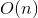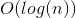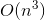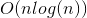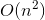Explanation:

Selection sort is comprised of outer and inner for loops that swap elements of the unsorted array into a sorted array. The largest possible number of times each loop can run is the number of elements in the array. Thus, the worst possible run time is.

### Example Question #1 : Sorting

True or False.

Selection sort is quicker than MergeSort.

True

False

False

Explanation:

MergeSort is has a running time of O(N). Selection sort has a running time of O(N2). Selection sort has O(N2) comparisons due to the swap in the algorithm.

### Example Question #1 : Selection Sort

What is the worst-case run-time of selection sort (in Big-O notation?)Explanation:

Selection sort is comprised of outer and inner for loops that swap elements of the unsorted array into a sorted array. The largest possible number of times each loop can run is the number of elements in the array. Thus, the worst possible run time is.

### Example Question #1 : Sorting

True or False.

Selection sort is quicker than MergeSort.

True

False

False

Explanation:

MergeSort is has a running time of O(N). Selection sort has a running time of O(N2). Selection sort has O(N2) comparisons due to the swap in the algorithm.

### Example Question #1 : Insertion Sort

Which of the following statements explains insertion sort?

All numbers less than the average are inserted on the left, and the rest is inserted at the right. The process is then repeated for the left and right side until all numbers are sorted.

The list is iterated through multiple times until it finds the desired first number, then repeats the process for all numbers.

It removes one element from the list, finds where it should be located, and inserts it in that position until no more elements remain.

None of these are insertion sort.

The list is broken apart into smaller lists that are sorted and merged back together.

It removes one element from the list, finds where it should be located, and inserts it in that position until no more elements remain.

Explanation:

Insertion sort removes an element from a list, checks the values adjacent to it to see if it is greater or smaller, until it finds the position where the number to the left is smaller and the number to the right is larger and places it there.

### Example Question #2 : Sorting

{1, 9, 5, 4, 2, 0, 4}

What would the set of numbers look like after four iterations of Insertion Sort?

{1, 4, 4, 5, 9, 2, 0}

{1, 4, 5, 9, 2, 0, 4}

{1, 4, 4, 9, 5, 2, 0}

{1, 9, 4, 5, 2, 0, 4}

{1, 4, 9, 5, 2, 0, 4}

{1, 4, 5, 9, 2, 0, 4}

Explanation:

Insertion Sort is a sorting algorithm that starts at the beginning of an array and with each iteration of the array it sorts the values from smallest to largest.

Therefore, after four iterations of Insertion Sort, the first four numbers will be in order from smallest to largest.

### Example Question #3 : Sorting

Which of the following do we consider when choosing a sorting algorithm to use?

I. Space efficiency

II. Run time efficiency

III. Array size

IV. Implementation language

I, II, III

II, III, IV

II, IV

I, II, III, IV

I, II

I, II, III, IV

Explanation:

All of the choices are important when choosing a sorting algorithm. Space and time complexity are the characteristics by which we measure the performance of an algorithm. the array size directly affects the performance of an algorithm. In addition, the language which the algorithm is written in can also affect the performance (for example, insertion sort may run faster in one language versus another, due the way the language was designed).

### Example Question #2 : Sorting

How is merge sort accomplished?

If there are an even number of elements, the list is broken into two groups, are sorted, then merged back together. If there are odd numbered elements, the list is broken into three groups.

The original list is continuously broken up into sublists until each sublist is containts 1 element, then the sublists are combined together.

The original list is broken into two groups, then sorted from there.

The orginal list is broken into sublists of 4, then are combined together.

Each element in the list is compared to all the other elements and inserted where it fits.

The original list is continuously broken up into sublists until each sublist is containts 1 element, then the sublists are combined together.

Explanation:

In merge sort, a list is broken up into sublists containing 1 element. Each element is then compared to another element and sorted. Each 2-element group is then combined with other 2-element groups, comparing the first value of each group and deciding how to four elements. The larger group of four is compared to another group of four, until the process ends and the list is sorted.

### Example Question #1 : Sorting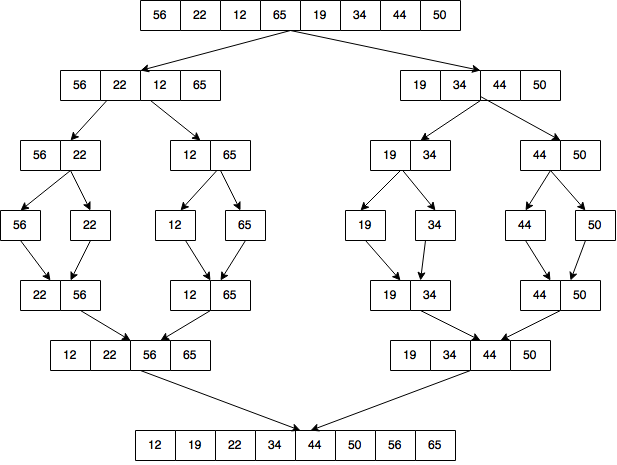The above diagram represents what type of sorting algorithm?

Bubblesort

Mergesort

Quicksort

Selection sort

Insertion sort

Mergesort

Explanation:

Mergesort consists of breaking down an unsorted list into subarrays; sorting each sub-array, and recombining the sub-arrays into larger arrays.

### Example Question #1 : Mergesort

Of the choices below, what is the most efficient sorting algorithm for an unordered list where the size of the list is an odd number and the size of the list is finite?

Bubble Sort

Insertion Sort

Mergesort

Selection Sort